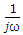### Circuit Theory and Control - MCQs from AMIE Exams (Winter 2018)

Choose the correct answer (10 x 2)

1. A certain current source has the values Is = 4μA and Rs = 1.2 MΩ. The values for an equivalent voltage source are
(a) 4.8 µV, 1.2 MΩ
(b) 1 V, 1.2 MΩ
(c) 4.8 V, 4.8 MΩ
(d) 4.8 V, 1.2 MΩ

2. In a two-source circuit, one source acting alone produces 12 mA through a given branch. The other source acting alone produces 10 mA in the opposite direction through the same branch. The actual current through the branch is
(a) 22 mA
(b) 12 mA
(c) 10 mA
(d) 2 mA

3. An RL low-pass filter consists of a 5.6 mH coil and a 3.3 kΩ resistor. The output voltage is taken across the resistor. The circuit's critical frequency is
(a) 93.8 kHz
(b) 93.8 Hz
(c) 861 Hz
(d) 86.12 kHz

4. A 15Ω resistor, a 220 µH coil, and a 60 pF capacitor are in series across an ac source. What is the bandwidth of the circuit?
(a) 138 MHz
(b) 10,866 Hz
(c) 1,907 Hz
(d) 138 kHz

5. Consider the following statements associated with two-port networks.
(a) Z12 = Z21
(b) Y21 = Y21
(c) h12 = h21
(d) AD - BC = 1

Which one of the statement given above is correct ?
(a) 1, 2, 3
(b) 2 ,3, 4
(c) 1, 3, 4
(d) 1, 2, 4

6. For the system in the given figure. The transfer function C(s)/R (s) is

(a) G1 + G2 + 1
(b) G1G2 + 1
(c) G1G2 + G2 + 1
(d) G1G2 + G1 + 1

7. In a two-phase ac servomotor rotor resistance is R and rotor reactance is X. The speed curve will be linear if
(a) X/R << 1
(b) X/R >> 1
(c) X/R = 1
(d) X2 = R

8. First column elements of Routh’s tabulation are 3, 5, -3/4, 1/2, 2. It means that there
(a) is one root in the left half s plane
(d) are two roots in the left half s plane
(c) are two roots in the right half s plane
(d) is one root in the right half s plane

9. When a unit step voltage drives a lag network the output
(a) remains constant at unit step value
(b) increases exponentially from zero to final value
(c) decreases exponentially from 1 to 0
(d) either (b) of (c) depending on Values of R and C

10. In the Bode diagram (log magnitude plot) the factor 1/jω in the transfer function gives a line having a slope,
(a) -20 dB per octave
(b) -10 dB per octave
(c) -6 dB per octave
(d) -2 dB per octave

1. (d)

I = 4 x 10-6 Amps.
R = 1.2 x 106 Ohms.
V = IR.
So, V = 4 x 10-6 x 1.2 x 106 = 4.8 V.
R = 4.8/4 x 10-6 = 1.2 MΩ.

2. (d) Algebraic sum of currents = 12 - 10 = 2mA

3. (a)

Freq. = R/(2πL)
= 3300/(2 x 3.1416 x 0.0056)
= 93,787 Hz or 93.8 KHz

4. (b)

BW = f/Q
Q = (1/R)√(L/C) = 127.65 (after putting values)
f = 1/2 x π x √(L/C) = 1385265.971 (After putting values)
f/Q = 10852

5. (d)

In two port network
Z12 = Z21
Y21 = Y21
h12 = - h21

6. (c)

Transfer function = (G1 + 1) G2 + 1 = G1 G2 + G2 + 1.

7. (a)

In a two-phase servomotor<< 1 so the torque-speed curve is almost linear.

8. (c)

Routh Hurwitz criteria are used to find the stability of the system and this is determined by the number of roots in which the number of roots is equal to the number of "sign" changes.

Here, the number of sign changes = 2

9. (b)

Because the lag network has R and C. The capacitor at t = 0 will be short and at t = infinity will become battery means an open circuit. Exponentially function describe this nature of capacitor for the lag network.

10. (c)

Forterm the slope is -20 dB/decade or -6 dB/octave because - 20 log 2 = -6 dB.

---
• The study material for AMIE/B Tech/Junior Engineer exams is available at https://amiestudycircle.com
• If you like the post please share your thoughts in the comment section